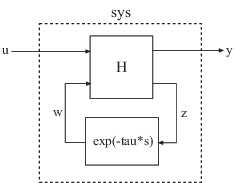# setDelayModel

Construct state-space model with internal delays

## Syntax

```sys = setDelayModel(H,tau) sys = setDelayModel(A,B1,B2,C1,C2,D11,D12,D21,D22,tau) ```

## Description

`sys = setDelayModel(H,tau)` constructs the state-space model `sys` obtained by LFT interconnection of the state-space model `H` with the vector of internal delays `tau`, as shown:`sys = setDelayModel(A,B1,B2,C1,C2,D11,D12,D21,D22,tau)` constructs the state-space model `sys` described by the following equations:

`$\begin{array}{c}\frac{dx\left(t\right)}{dt}=Ax\left(t\right)+{B}_{1}u\left(t\right)+{B}_{2}w\left(t\right)\\ y\left(t\right)={C}_{1}x\left(t\right)+{D}_{11}u\left(t\right)+{D}_{12}w\left(t\right)\\ z\left(t\right)={C}_{2}x\left(t\right)+{D}_{21}u\left(t\right)+{D}_{22}w\left(t\right)\\ w\left(t\right)=z\left(t-\tau \right).\end{array}$`

`tau` (τ) is the vector of internal delays in `sys`.

## Input Arguments

 `H` State-space (`ss`) model to interconnect with internal delays `tau`. `tau` Vector of internal delays of `sys`. For continuous-time models, express `tau` in seconds. For discrete-time models, express `tau` as integer values that represent multiples of the sample time. `A,B1,B2,C1,C2,D11,D12,D21,D22` Set of state-space matrices that, with the internal delay vector `tau`, explicitly describe the state-space model `sys`.

## Output Arguments

 `sys` State-space (`ss`) model with internal delays `tau`.

## Tips

• `setDelayModel` is an advanced operation and is not the natural way to construct models with internal delays. See Time Delays in Linear Systems for recommended ways of creating internal delays.

• The syntax `sys = setDelayModel(A,B1,B2,C1,C2,D11,D12,D21,D22,tau)` constructs a continuous-time model. You can construct the discrete-time model described by the state-space equations

`$\begin{array}{c}x\left[k+1\right]=Ax\left[k\right]+{B}_{1}u\left[k\right]+{B}_{2}w\left[k\right]\\ y\left[k\right]={C}_{1}x\left[k\right]+{D}_{11}u\left[k\right]+{D}_{12}w\left[k\right]\\ z\left[k\right]={C}_{2}x\left[k\right]+{D}_{21}u\left[k\right]+{D}_{22}w\left[k\right]\\ w\left[k\right]=z\left[k-\tau \right].\end{array}$`

To do so, first construct `sys` using ```sys = setDelayModel(A,B1,B2,C1,C2,D11,D12,D21,D22,tau)```. Then, use `sys.Ts` to set the sample time.

## Version History

Introduced in R2007a